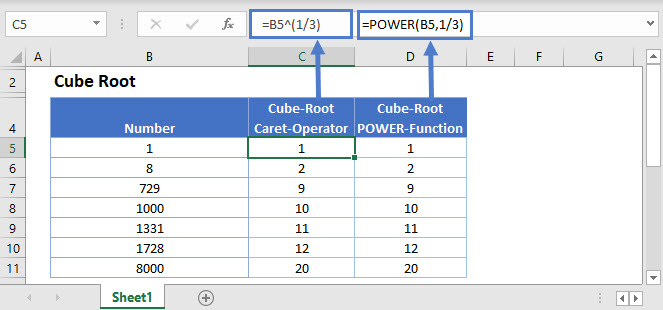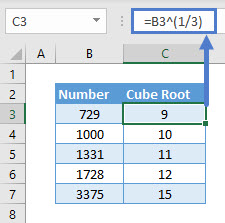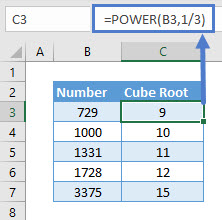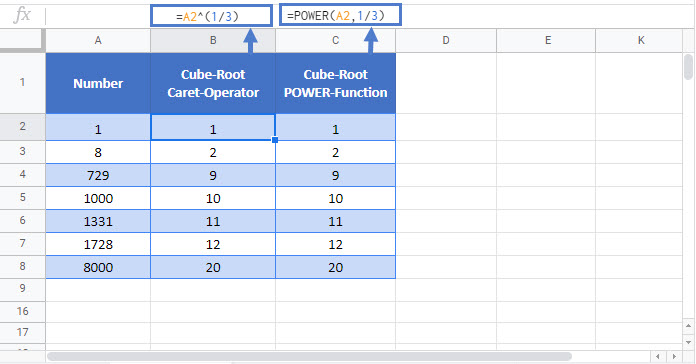# Cube Root Formula – Excel & Google Sheets

This tutorial will demonstrate how to find the cube root of a number in Excel & Google Sheets.<<master image>>

## Cube Root using Caret Operator (^)

To calculate the cube root of a number, you can use the Caret/Exponent (^) Operator or the POWER Function.

First, let’s see how to use the Caret Operator.

``=B3^(1/3)``The Caret Operator raises a number to a power. By raising, to 1/3 we take the cube root of a number (or the nth root).

Note: To control the order of operations make sure the exponential ‘1/3’ is in parentheses.

## Cube Root using POWER Function

The POWER function returns the result for a given number raised to a power. Its syntax is:

``=POWER(B3,1/3)``In order to find the cube root, make sure you enter 1/3 and not 3.

Note: Same process can be used to calculate the cube root of a negative number.

## Cube Root in Google Sheets

These formulas work exactly the same in Google Sheets as in Excel.### Excel Practice Worksheet

Practice Excel functions and formulas with our 100% free practice worksheets!